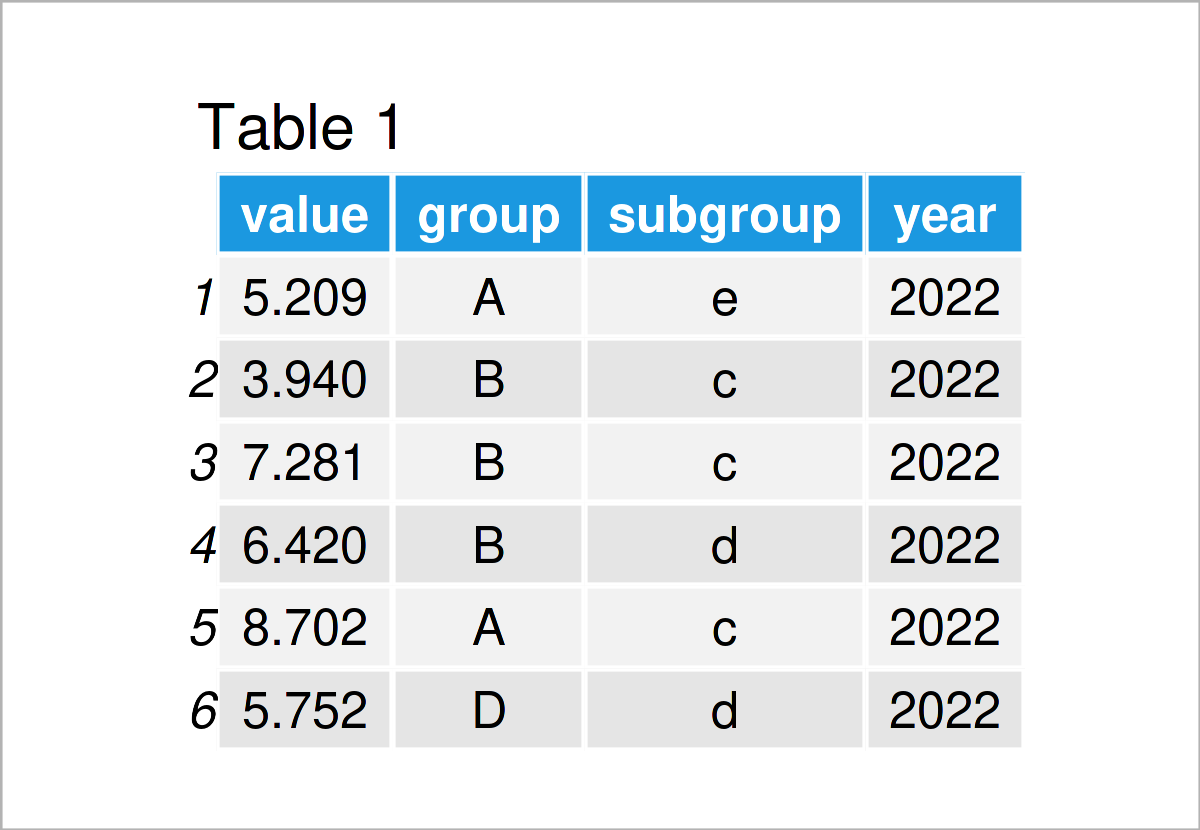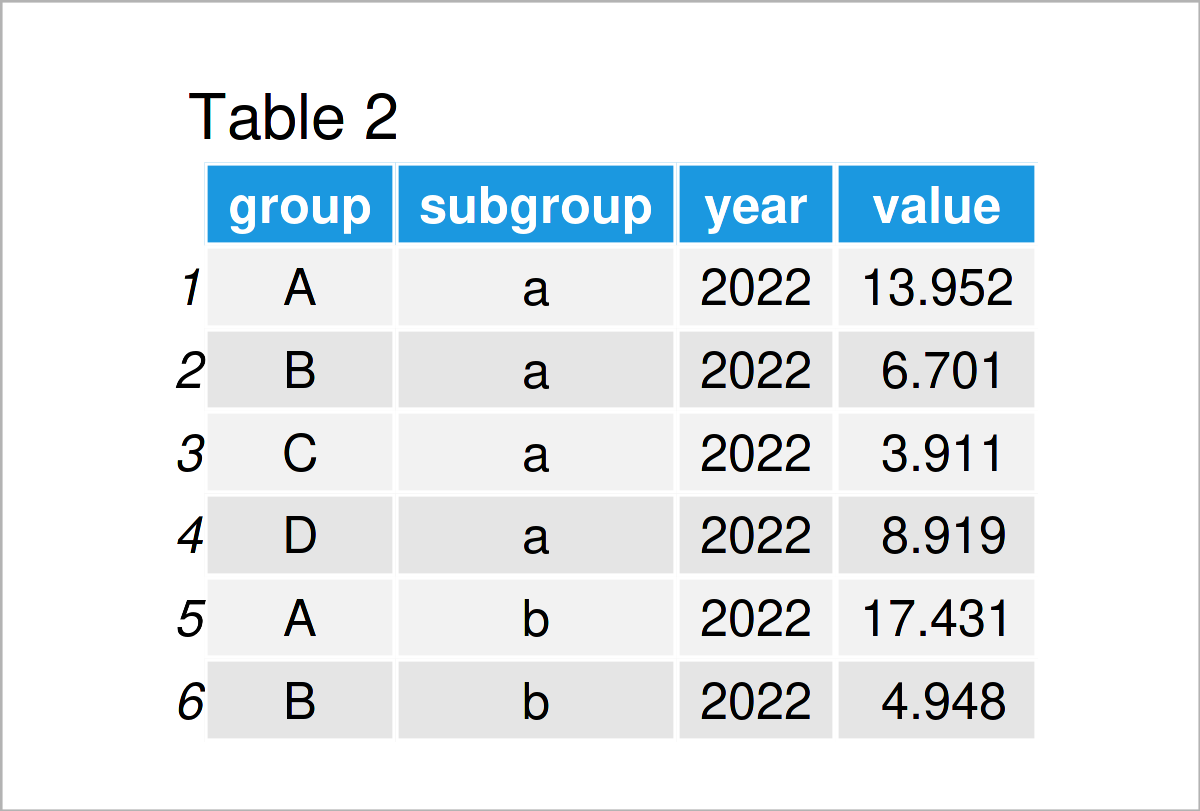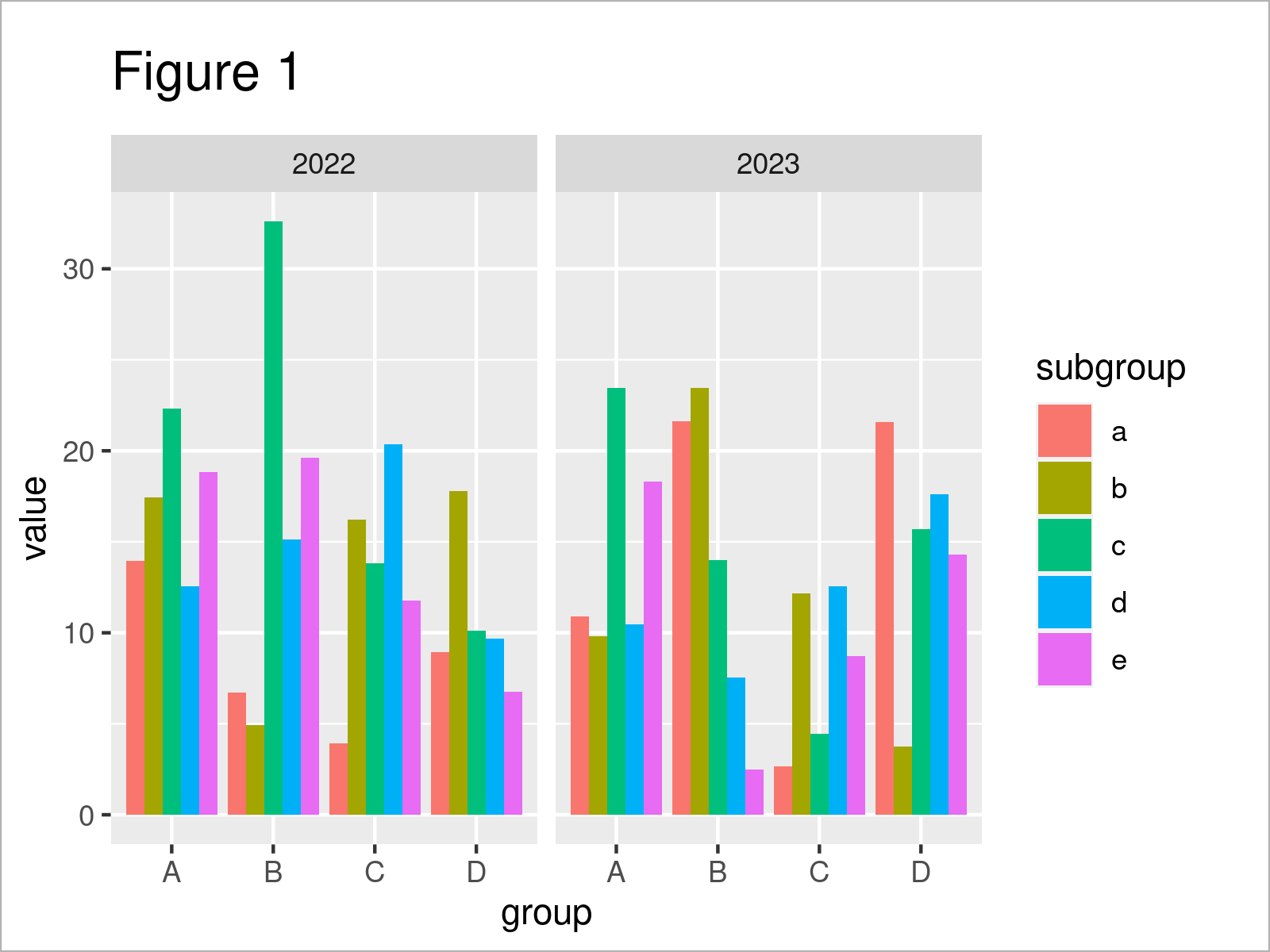# Plot Two Categorical Variables on X-Axis & Continuous Data as Fill in R (Example)

This article demonstrates how to draw two categories on the x-axis and multiple other variables as fill in R programming.

The content looks as follows:

Let’s get started…

## Example Data

Consider the following example data:

```set.seed(349476)                                         # Create example data frame
data <- data.frame(value = rnorm(100, 5, 2),
group = sample(LETTERS[1:4], 100, replace = TRUE),
subgroup = sample(letters[1:5], 100, replace = TRUE),
year = c(rep(2022:2023, each = 50)))Table 1 shows the first six lines of our example data: Furthermore, you can see that our example data has four columns.

The variable value has the numeric class. This variable contains all our continuous data.

The variables group and subgroup are character strings, and the variable year has the integer class. Those other variables are used to group our continuous data into different subcategories.

As a next step for the preparation of our data, we have to decide what we want to measure. In this specific example, I’ll explain how to calculate the sum for each of our groups.

In order to calculate the sum by group, we can use the aggregate function as demonstrated below:

```data_aggr <- aggregate(value ~ group + subgroup + year,  # Calculate sum by group
data,
sum)
head(data_aggr)                                          # Print aggregated data frame```As shown in Table 2, the previous R code has created a new data frame called data_aggr. This data frame contains a single value for each of our subgroups in each of our years.

Let’s draw these data!

## Example: Draw Multiple Categorical Variables on X-Axis & Continuous Data as Fill

This section shows how to create a graphic that splits our data into two main categories on the x-axis, as well as into groups and subgroups within each of those categories.

We’ll use the ggplot2 package to draw our data. To be able to use the functions of the ggplot2 package, we first have to install and load ggplot2.

```install.packages("ggplot2")                              # Install ggplot2 package

In the next step, we can use the ggplot, geom_col, and facet_wrap functions to visualize our data:

```ggplot(data_aggr,                                        # Draw ggplot2 plot
aes(x = group,
y = value,
fill = subgroup)) +
geom_col(position = "dodge") +
facet_wrap(year ~ .)```In Figure 1 you can see that we have created a new ggplot2 plot by running the previous code. This plot contains our two years in two separate facets. Each of these facets contains a grouped barplot, where we have used the column group on the x-axis and the column subgroup to separate the bars within each main group.

This kind of plot can be very useful when you want to illustrate data with multiple subgroups over several years.

## Video, Further Resources & Summary

I have recently released a video on my YouTube channel, which shows the contents of this tutorial. You can find the video below:

Besides that, you might read some of the other tutorials on https://statisticsglobe.com/. I have published several tutorials already.

At this point you should have learned how to plot two categories on the x-axis and multiple other variables as fill in the R programming language. Let me know in the comments, in case you have further questions.

Subscribe to the Statistics Globe Newsletter